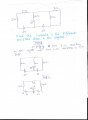# Currents in different parts of resistor

#### logearav

Joined Aug 19, 2011
243
Please refer my attachments, revered members.
In Step 2 the 4 ohm resistor is removed, its been mentioned that no current will go through any resistor. Why? Still current can flow through the 4 ohm resistor that is connected in the right extreme end, can't it?
Again, it has been mentioned that if 4 ohm resistor is added between d and e, no current will be drawn into it. Can i know why?#### DickCappels

Joined Aug 21, 2008
10,067
Current through a resistor equals the voltage across the resistor divided by the resistor's resistance. Since both e and d are at the same voltage, no current would flow through a resistor connected across those points in the circuit..

#### Jony130

Joined Feb 17, 2009
5,457
Please do the circuit analysis and solve the circuit and then you will know why.

#### logearav

Joined Aug 19, 2011
243
Current through a resistor equals the voltage across the resistor divided by the resistor's resistance. Since both e and d are at the same voltage, no current would flow through a resistor connected across those points in the circuit..
I = V/R. The extreme left resistor of resistance 4 ohm is connected to voltage of 2V. So current is 2/4 that gives 0.5 A of current. How then we conclude no current is flowing through any of the resistor?

#### Jony130

Joined Feb 17, 2009
5,457
Please notice that Va = 2V and Vc = 2V. So the Left and the right resistor will see the difference in this voltage.
Vd = Va - Vc = 2V - 2V = 0V; And I = Vd/(4Ω + 4Ω) = 0V/8Ω = 0A

#### logearav

Joined Aug 19, 2011
243
Please notice that Va = 2V and Vc = 2V. So the Left and the right resistor will see the difference in this voltage.
Vd = Va - Vc = 2V - 2V = 0V; And I = Vd/(4Ω + 4Ω) = 0V/8Ω = 0A
Thanks sir.
But the point d is connected to the positive terminal of the battery and point b is to negative terminal. If we assume the potential at b to be zero then Vd is 2-0 so 2V. How it is zero as mentioned by you, sir?

#### Jony130

Joined Feb 17, 2009
5,457
Sorry for confusion. I did not notice that you already have Vd on the diagram.
So let as start again
The voltage difference between point a and b is equal to :
Vac = Va - Vc = 2V - 2V = 0V
And this means that no current can flow between point a and c. So, no current in left and right resistor.
I = Vac/(4Ω + 4Ω) = 0V/8Ω = 0A
If this is the case the voltage at point e is also equal to 2V
Ve = Va - I*4Ω = 2V - 0A*2Ω = 2V
And the middle resistor current is Im = (Ve - Vd)/Rm = (2V - 2V)/4Ω = 0A

#### logearav

Joined Aug 19, 2011
243
The voltage difference between point a and b is equal to :
Vac = Va - Vc = 2V - 2V = 0V

Thanks again sir.
Voltage difference between a and b should be Vab, Am i right or as mentioned by you i.e Vac?

#### Jony130

Joined Feb 17, 2009
5,457
The voltage difference between point a and b is equal to :
Vac = Va - Vc = 2V - 2V = 0V

Thanks again sir.
Voltage difference between a and b should be Vab, Am i right or as mentioned by you i.e Vac?
I meant to say: the voltage difference between point a and c is equal to : Vac

•logearav

#### WBahn

Joined Mar 31, 2012
29,149